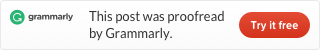# LeetCode problem 992 Subarrays with K Different Integers (Java)

Given an array `A` of positive integers, call a (contiguous, not necessarily distinct) subarray of `A` good if the number of different integers in that subarray is exactly `K`.

(For example, `[1,2,3,1,2]` has `3` different integers: `1``2`, and `3`.)

Return the number of good subarrays of `A`.

Example 1:

Input: A = [1,2,1,2,3], K = 2
Output: 7
Explanation: Subarrays formed with exactly 2 different integers: [1,2], [2,1], [1,2], [2,3], [1,2,1], [2,1,2], [1,2,1,2].

Example 2:

Input: A = [1,2,1,3,4], K = 3
Output: 3
Explanation: Subarrays formed with exactly 3 different integers: [1,2,1,3], [2,1,3], [1,3,4].

Note:

1. `1 <= A.length <= 20000`
2. `1 <= A[i] <= A.length`
3. `1 <= K <= A.length`

This problem is very hard if you want to use one sliding window to solve it. But we can use two sliding windows to make it easy to solve.

First, we can easily to implement a sliding window to get a result of the number of different integers in that subarray is equal to or smaller than K.

Then subarraysWithMostKDistinct(k) – subarraysWithMostKDistinct(k-1) will get the result of the number of different integers in that subarray is exactly K.

Then we got the final function subarraysWithKDistinct.

And aonther approach.